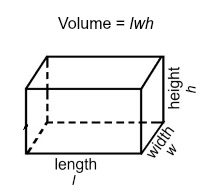# Question #17019

Mar 23, 2017We have been given a cuboid having

$\text{length } \left(l\right) = 8 c m = 0.08 m$

$\text{height } \left(h\right) = 6 c m = 0.06 m$

$\text{width } \left(w\right) = 4 c m = 0.04 m$

and

$\text{weight} \left(W\right) = 100 N$

It is obvious that two surfaces having smallest area comprising height and width will have area $A = h \times w = 0.06 \times 0.04 {m}^{2} = 24 \times {10}^{-} 4 {m}^{2}$

When the cuboid is kept on the table keeping its surface of minimum area on it then the pressure (force exerted on unit area perpendicularly) on the table by its weight will be maximum and this will be given by

${P}_{\text{max"=W/A=100/(24xx10^-4)N"/}} {m}^{2}$

$= 4.16 \times {10}^{4} P a$